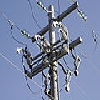#### You may also like### Differential Equation Matcher

Match the descriptions of physical processes to these differential equations.### The Real Hydrogen Atom

Dip your toe into the world of quantum mechanics by looking at the Schrodinger equation for hydrogen atoms### Differential Electricity

As a capacitor discharges, its charge changes continuously. Find the differential equation governing this variation.

# The Not-so-simple Pendulum 2

##### Age 16 to 18Challenge Level

A simple pendulum released from rest from an angle $y$ of less that $20^\circ$ is well-modelled by the linear differential equation
$$m\frac{d^2 y}{dt^2} +k^2y=0\;.$$
Can you work out the meaning of the various letters in the equation, the units of the constant $k$, and find the general solution to this equation?

Look at the solution: it keeps on oscillating for ever. Clearly, this is an unreasonable assumption, as real pendulums stop swinging due to friction.

Here friction is modelled by a force term which is directly proportional to the velocity of the object, acting in the opposite direction to the motion. Do you think that this is a good modelling assumption? What are its strengths and weaknesses?

With this assumption, the differential equation becomes
$$m\frac{d^2 y}{dt^2} + \lambda \frac{d y}{dt}+k^2y=0\;.$$
Can you see how this equation satisfies all of requirements of a motion under friction? What are the units of $\lambda$, and what features of the motion would contribute to it in practice? It is assumed that $\lambda$ is constant: is this a reasonable assumption?

Although this equation might look complicated it is still only a second order differential equation with constant coefficients, so can be solved using standard methods (and a lot of algebra) to give
$$y = \exp\Big[-\frac{\lambda t}{2m}\Big]\Big[A\cos(\Lambda t)+\sin(\Lambda t)\Big] \quad\mbox{and}\quad \Lambda = \sqrt{\frac{k^2}{m}-\frac{\epsilon^2}{4m^2}}\;.$$

Test your skills of differentiation by showing that this equation indeed satisfies the differential equation. More importantly, analyse the solution: how well does it model friction? Consider in particular the amplitude and the frequency of the damped oscillation. Does this meet with your intuitive expectation?